## Random Posts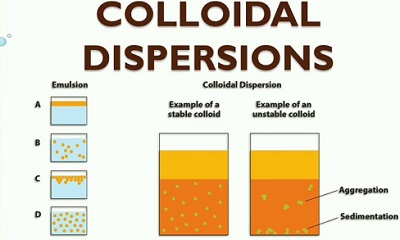## Preparation of Colloids (Class 12)

Preparation of Colloidal Solution of Starch, Arsenious Sulphide, Ferric Hydroxide (Class 12)

Colloidal solutions where the dispersed phase is a solid and the dispersion medium is a liquid are generally called sols. Sols are divided into two types:

1. Lyophilic sols

2. Lyophobic sols

In lyophilic sols, there is greater affinity between the dispersed phase and the dispersion medium, and hence they are more stable. But in lyophobic the affinity is less and hence they are less stable. FDor the same reason lyophilic sols can be prepared more easily by simply shaking or warming the substance with the dispersion medium. But preparation of lyophobic sols require special methods.

Examples of lyophilic sols: Starch, gelatin etc.

Examples of lyophobic sols: As2S3, Fe(OH)3, Al(OH)3, colloids of metals like Au, Ag, Pt etc.

1. Preparation of Starch sol

About 2g of starch powder is mixed with water and made into a thin paste in a clean china dish. This is then added to about 100 mL of hot water taken in a 250 mL beaker, while stirring with a glass rod. The content is boiled for a few minutes stirring all the time. The cooled solution is then filtered through a filter paper to separate any undissolved matter. The filtrate obtained is starch sol.

2. Preparation of Arsenious Sulphide (As2S3) sol

As2O3 + 3H2S As2S3 + 3H2O

About 1.5g of As2S3 in about 200mL of water is taken in a beaker. It is then boiled for about 15 minutes, stirring all the time with a glass rod. Then cool the solution to room temperature and filter through a filter paper to remove any undissolved As2O3. Transfer the filtrate in to a 500 mL conical flask and pass H2S gas till the solution is saturated with H2S.

An yellow orange coloured As2S3 is formed. The solution is filtered and the filtrate is a sol of As2S3.

3. Preparation of Ferric Hydroxide (Fe(OH)3) sol

FeCl3 + 3H2O →Fe(OH)3 + 3HCl

About 250mL of water is taken in a beaker and heated to boil. To the boiling water, add about 25mL 2% FeCl3 solution. It is boiled further to get a dark brown coloured solution. The solution is then dialysed to remove the HCl produced by the hydrolysis of FeCl3.

Dialysis

A simple type of dialyser consists of a parchment or cellophane bag. Tie the top of the bag round the sleeve of a wide bore glass tube by means of a string. Fill the bag with the prepared sol to be dialysed. Suspend the bag in a large beaker containing distilled water and leave for about 24 hrs. The electrolyte impurities pass through the bag while the colloidal solution is held back.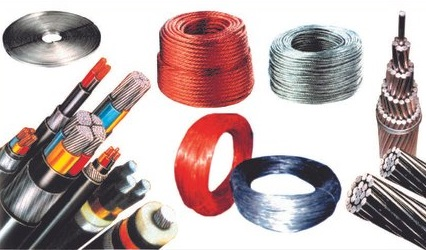## Characteristics of Conductor Materials

Characteristics of Conducting Materials

1. Conductivity (σ) :

The reciprocal of the material's electrical resistance is the conductivity (σ). Mhos/cm is the unit of conductivity. It is the property of a material that allows electric current to flow freely through it. In other words, it makes it simple for electric current to pass through the material.

2. Tensile Strength :

The ability to resist load without failure is characterised as a material's strength. The ability of a material to sustain a stretching (tensile) force without fracture is referred to as tensile strength. As a result, tensile strength indicates the conductor limit, beyond which significant distortion or fracture occurs. It's measured in load per square metre of cross-sectional area. (tonnes / cm2).

3. Ductility :

It refers to a material's capacity to deform plastically under tensile load without rupturing. A ductile substance can be pulled into a fine wire and bent, twisted, or modified in shape without breaking. Difficult materials include gold, silver, copper, aluminium, nickel, tin, and lead.

4. Corrosion Resistance :

The electrochemical attack causes corrosion, which is a slow process in a material. The metal is generally converted into an oxide, salt, or some other compound as a result of the chemicals present in the atmosphere and if the material is exposed, the metal is generally converted into an oxide, salt, or some other compound, and thus the metal does not serve the purpose it was intended to serve. It can also happen at high temperatures in materials that are inert at room temperature or below.

5. Effect of Alloying on Resistivity :

Resistivity is increased by any impurity, whether metallic or non-metallic. The influence of metal impurities on a metal's resistivity is determined by the type of alloy produced. When the atomic volumes and melting temperatures of the two metals differ significantly, the alloy is made up of crystals from both metals. Mechanical mixes are the name given to such alloys. In these alloys, the resistivity and temperature coefficient of resistivity fluctuate linearly with the degree of impurity content.

When the atomic volumes of the two metals differ by less than 15%, the alloy has a single crystal structure in which the atoms of both metals are accommodated by the crystal lattice. Solid solution alloy is the name for this type of alloy. With a particular level of impurity content, the resistivity increases and the temperature co-efficient falls in this type of alloy. If the impurity is increased after that, the resistivity decreases and the temperature co-efficient increases.

Chemically mixed alloys are created when metals combine chemically. The relationship between resistivity and temperature coefficient of resistivity and impurity content is intricate. The alloying increases the mechanical strength of the material and makes it harder.

6. Alloying Effect on Mechanical Properties:

The addition of even a little amount of some alloys increases the mechanical and physical properties of the material. The following are a few of the outcomes:

(a) Copper: It improves ductility while increasing strength and hardness. Corrosion resistance is improved as well.

(b) Aluminium: It serves as a deoxidizer, slowing grain development and facilitating nitriding.

(c) Lead: It makes things easier for the machine.

(d) Nickel: It improves tensile strength while keeping ductility. The coefficient of thermal expansion is reduced as toughness increases. Reduces corrosion while increasing hardness marginally.

(e) Tungsten: A high carbide forming propensity slows grain formation and enhances cohesive force significantly.

(f) Silicon: Enhances magnetic permeability as well as electrical resistance. Increases the ability to resist oxidation. Increases ultimate strength. Assists in the strengthening of ferrite.

(g) Chromium: Strengthens and hardens without compromising ductility. Wear and corrosion resistance are improved. Improves toughness and raises the critical temperature.

(h) Manganese: Reduces the melting point of iron, lowers the critical temperature, and promotes steel hardenability.

(i) Cobalt: Enhances the toughness of high-speed steel by providing good magnetic characteristics.

7. Solderability :

A fusible alloy named solder is used to bond metal surfaces. The property comes particularly handy when two metal objects need to be linked together, such as when wires are involved.

8. Brittleness:

Metal tends to break when struck with a hammer, and it is the polar opposite of toughness. Shock loads are easily broken by the fragile material.

Stranding of Conductors

A large cross-section of a conductor gets rigid. During handling, it is prone to kinking and breaking. Conductors are stranded to prevent this. Stranded conductors are lightweight and easy to coil. The layers of a stranded conductor are formed by twisting small wires called strands together. Each layer's wires are helical-wrapped around the layer before them. For each layer, stranding is done in the opposite direction. If the wires in one layer are twisted left to right, the wires in the following layer will be twisted right to left, and so on. A central wire or strand is frequently twisted around which additional layers of strands are wound.

6 wires wrap around 1 wire, 12 wires wrap around the preceding 6, 18 wires wrap around 12, 24 wires wrap around 18, and so on. The number of layers available will be determined by the number of wires available. A layer isn't counted if the centre wire isn't present. Three or four-stranded wires can be placed in the centre instead of a single wire, and layers can be constructed over them. If you add two stranded wires in the middle, the first layer will have 9 wires, the second layer will have 15, and so on. The number of wires in each consecutive layer increases by 6 in each of the examples above. The number of wires in each layer, the total number of wires, and the overall diameter of the stranded conductor are shown in the table below.

Details of Stranded Conductor

 No: of wires in the centre 1 wire 3 wires 4 wires The number of wires in the nth layer from the centre 6n 3 + 6n 4 + 6n The total number of wires in an n-layer stranded conductor 1 + 3n ( 1 + n) 3 (1 + n)2 (4 + 3n) ( 1 + n) Diameter in centimetres over the nth layer, where d is the diameter of each wire in centimetres. (1 + 2n) d (2.155 + 2n) d (2.414 + 2n) din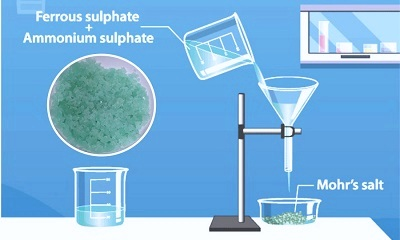## Preparation of Double Salts (Class 12)

1. Preparation of Double Salt - Preparation of Mohr’s Salt

Mohr’s Salt is Ferrous Ammonium Sulphate. FeSO4(NH4)2SO4.6H2O. It is a double salt of ferrous sulphate and ammonium sulphate. It is prepared by mixing solutions of ferrous sulphate and ammonium sulphate containing equimolar proportions of the two substances.

Procedure

A solution containing 24g of FeSO4.7H2O in 100mL of water is mixed with a solution of 11.4g of (NH4)2SO4 in 100mL of water. The mixture is heated until its volume is reduced almost to half and then it is filtered hot. The clear solution is set aside in an evaporating dish. Light green crystals of ferrous ammonium sulphate [FeSO4(NH4)2SO4.6H2O] will be deposited. It is filtered at the pump. The crystals are washed with a little distilled water and then dried in a current of air.

Ferrous ammonium sulphate is a light green crystalline substance. It is stable to oxidation and is not dehydrated on standing. So its is preferred to FeSO4.7H2O which is efflorescent and is liable to oxidation by atmospheric air.

2. Preparation of Double Salt - Preparation of Potash Alum

Potash alum is potassium aluminium sulphate, or K2SO4 Al2(SO4)3 24H2O. It is a double salt and is usually called alum and is prepared by mixing solutions containing equimolar proportions of K2SO4 and Al2(SO4)3

Procedure

6g of K2SO4 in 100 mL of water is mixed with 23g of Al2(SO4)3. 18H2O in 100mL of water. The solution is heated, evaporated to almost half the volume and then filtered hot. The clear solution is kept in an evaporating dish. Crystals of the alum will be deposited. It is filtered and then washed at the pump with a little distilled water and then it is dried in a current of air. It is a colourless octahedral shaped substance. It is used for the purification of water and as a mordant.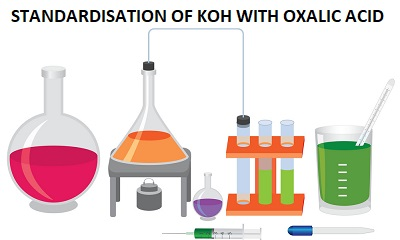## Standardization of KOH with Oxalic Acid

STANDARDISATION OF KOH (POTASSIUM HYDROXIDE) WITH OXALIC ACID

Determine the normality of the given solution of KOH using standard oxalic acid solution containing 6.2 gL-1

Principle:

The determination of normality is based on the reaction between KOH and oxalic acid as shown by the equation given below.

2KOH + H2C2O4  K2C2O4 + 2H2O

Here one mole of oxalic acid (two equivalents) reacts with two moles of KOH. Therefore, the equivalent mass of KOH is the same as its molecular mass (56).

Equivalent Mass of Oxalic acid = Molecular Mass/2 = 126/2 = 63

Equivalent Mass of KOH = Molecular Mass = 56

A known volume of KOH solution is titrated against the standard oxalic acid solution using phenolphthalein as the indicator. From the titre values, the normality and the mass per litre of KOH are calculated.

 Indicator End point In burette In pipette Phenolphthalein Pink to colourless Oxalic acid solution Potassium hydroxide solution

Procedure:

20 mL of the potassium hydroxide solution is pipetted out into a clean conical flask after rinsing the pipette with the same solution. One or two drops of phenolphthalein are added to it. It is then titrated against oxalic acid taken in the burette. The addition of acid is continued till the colour of the solution fades. The sides of the conical flask are washed with small quantities of water using a wash bottle. The titration is continued by adding acid in drops till the pink colour just disappears with a last single drop of acid. The end point is the disappearance of the pink colour. The titration is repeated to get concordant values. The results are tabulated as shown below. From the values, the normality and the mass per litre of KOH are calculated.

KOH x H2C2O4

 Expt. No: Volume of KOH Burette reading Volume of H2C2O4 Initial Final 1 2 3 20 20 20

Calculation

Volume of KOH solution, V1 = 20 mL

Let normality of KOH solution = N1

Normality of H2C2O4, N2 = Mass per litre/Eq. Mass = 6.2/63 = 0.0984

Let volume of H2C2O4 = V2 mL

N1 x V1 = N2 x V2

Normality of KOH, N1 = V2 x 0.0984/20

Mass per litre of KOH = N1 x 56g

Result

Normality of KOH = _____

Mass per litre of KOH = _____ g

NOTE:

Acidimetry: Acidimetry is the estimation of an alkali solution using a standard acid solution.

Alkalimetry: Alkalimetry is the estimation of an acid solution using a standard alkali solution. When an acid reacts with a base neutralization reaction takes place resulting in the formation of a salt and water. If both acid and base are strong then the resulting solution at the end point is neutral with pH = 7. On the other hand, if the acid or base is weak then the salt formed by the neutralization will undergo hydrolysis and the solution at the end point will be slightly alkaline or acidic. The indicators used in acidimetry and alkalimetry are phenolphthalein and methyl orange. These indicators are weak organic acids or bases. In acid-base titrations, acid should be taken in the burette and the base should be pippetted out into a conical flask.

A few other experiments in acidimetry and alkalimetry,

1. Determine the normality and the mass per litre of nitric acid using a standard solution of sodium hydroxide containing 4.1 gL-1.

2. Determine the strength and the mass per litre of sulphuric acid. You are provided with a standard solution of potassium hydroxide containing 5.5 gL-1.

3. Determine the normality and mass per litre of sodium carbonate. You are provided with hydrochloric acid solution containing 3.7 gL-1.

4. Determine the mass per litre of hydrochloric acid. You are supplied with a standard with a standard solution of potassium carbonate containing 7.0 gL-1.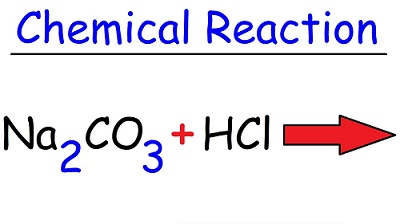## Standardization of Hydrochloric Acid using Sodium Carbonate

Standardization of Hydrochloric Acid using Sodium Carbonate

Determine the normality of hydrochloric acid using standard sodium carbonate solution containing 5.1 gL-1

Principle:

The determination of normality is based on the reaction between sodium carbonate and hydrochloric acid as follows.

Na2CO3 +2HCl => 2NaCl + H2O + CO2

It is clear from the equation that one mole of sodium carbonate (two equivalents) requires two moles of hydrochloric acid for complete reaction. Therefore, the equivalent mass of hydrochloric acid is same as its molecular mass.

Eq. mass of Na2CO3 = Molecular Mass/2 = 106/2 = 53

Eq. mass of HCl = Molecular Mass = 36.5

A known volume of standard sodium carbonate solution is titrated against the given hydrochloric acid using methyl orange as indicator. From the titre value, the normality and the mass per litre of hydrochloric acid are calculated.

 Indicator Methyl orange End point Yellow to pale orange red In burette HCl solution In pipette Na2CO3 solution

Procedure:

A clean burette is rinsed and then filled with the acid solution without air bubbles. A clean pipette is rinsed with the sodium carbonate solution. 20 mL of the standard sodium carbonate solution is then pipette out into a conical flask. One or two drops of methyl orange indicator are added to it to impart a golden yellow colour to the solution. It is then titrated against hydrochloric acid taken in the burette.

Wash the sides of the conical flask with water using a wash bottle near the end point. At the end point, the colour of the solution changes from golden yellow to pale orange red. The titration is repeated to get concordant values. The titre values are tabulated as shown below. From the values, the normality and the mass per litre of hydrochloric acid are calculated.

Na2CO3 x HCl

 Exp No: Volume of Na2CO3 Burette reading Volume of HCl used Initial Final 1 2 3 20 20 20

Calculation:

Volume of sodium carbonate solution, V1 = 20 mL

Normality of Na2CO3 solution, N1 = Mass per litre/Eq. Mass = 5.1/53 = 0.0962

Let volume of hydrochloric acid = V2 mL

Let normality of hydrochloric acid = N2

N1 x V1 = N2 x V2

Therefore, Normality of HCl, N2 = 0.0962 x 20/ V2

Mass per litre of HCl = N2 x 36.5 g

Result:

Normality of HCl = _______

Mass per litre of HCl = _______ g